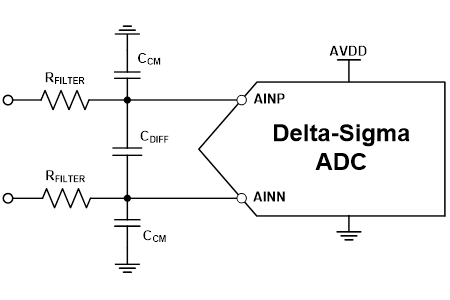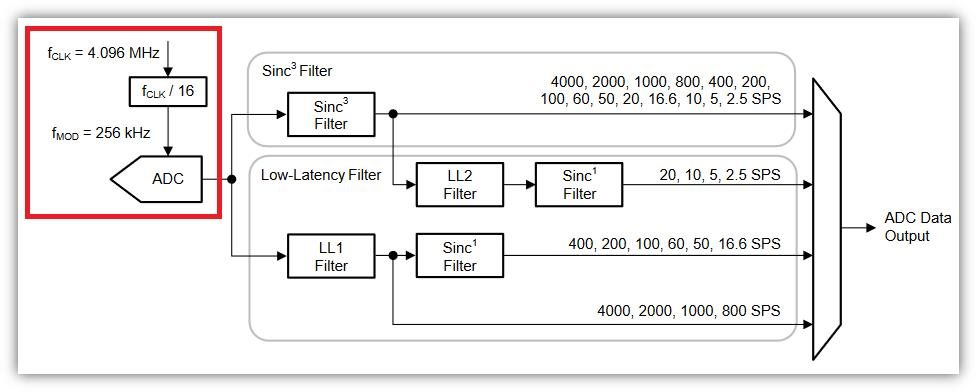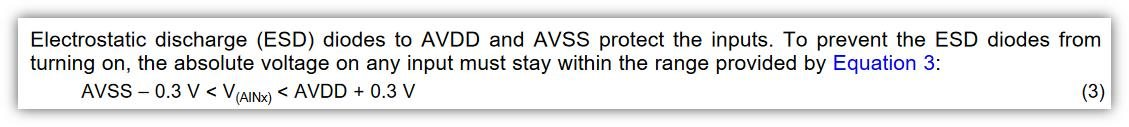If you have a related question, please click the "Ask a related question" button in the top right corner. The newly created question will be automatically linked to this question.

• Resolved

# [FAQ] Delta-sigma ADC anti-aliasing filter component selection

I am trying to develop a system using a low speed delta-sigma ADC for DC-type measurements. How do I select the component values for the anti-aliasing filter?

• Hello,

Low-speed delta-sigma ADCs generally require a simple single-pole RC filter to reduce aliasing effects. For differential signals, the filter structure is typically comprised of two filtering paths: a differential filter derived from the combination of both filter resistors, RFILTER, and the differential capacitor, CDIFF; and a common-mode filter derived from combination of one filter resistor, RFILTER, and the common-mode capacitor, CCM. This is shown in Figure 1.Figure 1: anti-aliasing filter structure for low-speed delta-sigma ADC

[NOTE: if you had a single-ended input where AINN is reference to ground, the filter would be comprised of RFILTER and CCM. However, the design guidelines would be the same as those for the differential filter described below.]

To determine the values of each component in Figure 1, it helps to break your analysis into three parts:

• What should the differential filter cutoff frequency be?
• What size filter resistor should I choose?
• What size differential and common-mode capacitors should I choose?

The first two questions can actually be answered in parallel since their answers do not depend on each other. The third question can be answered with the results of the first two. Moreover, for each question, the ADS124S08 – a 24-bit, 12-ch, 4-kSPS delta-sigma ADC – will also be used as an example data converter to help illustrate how to put these design principles into practice.

What should the differential filter cutoff frequency be?

Recall that the anti-aliasing filter's purpose is to keep frequency content at or near the ADC’s modulator frequency, fMOD, from aliasing back into the passband, since these frequencies are not natively rejected by the digital filter. As a result, set the differential filter’s 3-dB cutoff frequency, fC-DIFF, such that it is 10x to 100x lower compared to fMOD. This results in 20-dB to 40-dB rejection of frequencies around fMOD, respectively. The amount of rejection required depends on your system’s design goals. If you would like to learn more about anti-aliasing filter fundamentals as well as why you should be concerned about the modulator frequency aliasing, please check out our Precision Labs content on this topic (modules 4.5 and 4.6)

For the ADS124S08, fMOD is fCLK / 16, where fCLK is the master clock frequency as shown in Figure 2. Given the nominal internal oscillator frequency of 4.096 MHz, fMOD = 4.096 MHz / 16 = 256 kHz. Therefore, for this specific ADC, you would choose fC-DIFF = 2.56 kHz or fC-DIFF = 25.6k Hz to get 20 dB or 40 dB of rejection, respectively. Additionally, ensure that fC-DIFF is also greater than the digital filter's -3dB frequency, f3dB, or the RC filter can influence the digital filter characteristic.Figure 2: ADS124S08 digital filter structure and modulator clock

What size filter resistor should I choose?

In the system shown in Figure 1, the filter resistor also acts as a current limiter. As such, this resistor is sized to limit the maximum pin input current (IMAX) as shown in the ADC’s Absolute Maximum Ratings table. To determine the allowable voltage drop across this resistor, you will need the expected overvoltage conditions seen at the system input (VOV) as well as the turn-on voltage for the ADC’s integrated ESD protection diodes (VESD). Then, you can use the following equation to solve for the resistor value, RFILTER:

RFILTER > (VOV – VESD) / IMAX

For the ADS124S08, IMAX is 10mA as shown in table 7.1 in the device datasheet. Moreover, as Figure 3 shows, the ADS124S08’s ESD diodes turn on when the input voltage is 300mV beyond the analog supplies.Figure 3: ADS124S08 ESD information

If you assume AVDD = 5 V and that the maximum overvoltage condition you can expect, VOV, is 20 V, you now have all of the information necessary to determine the minimum size for RFILTER:

VOV = 20 V

VESD = AVDD + 0.3 V = 5.3 V

IMAX = 10 mA

RFILTER > (VOV – VESD) / IMAX = (20 V – 5.3 V) / 10 mA = 1,470 Ω

Note that this is the absolute smallest value the resistor can be to limit the current into the ADC pins given the system parameters. It is best practice to allow margin on the overvoltage conditions and the maximum current when calculating the resistor size. This ensures a more robust protection circuit that can accommodate any potential system variation. For example, you could assume a 10% tolerance on VOV as well as a 30% tolerance on IMAX:

VOV’ = VOV*1.1 = 22 V

VESD = AVDD + 0.3 V = 5.3 V

IMAX’ = IMAX*0.7 = 7 mA

RFILTER’ > (VOV’ – VESD) / IMAX’ = (22 V – 5.3 V) / 7 mA = 2,386 Ω

Once you calculate the appropriate resistor value, choose a standard resistor that is equal to or greater than this value.

What size differential and common-mode capacitors should I choose?

Since you have determined the filter cutoff and resistor size, you can use following equation to determine the size of the capacitor for the differential filter, CDIFF:

CDIFF = 1 / [2*π*fC*(2*RFILTER)]

The common-mode capacitors, CCM, are then chosen to be 10x to 20x smaller than CDIFF, such that:

CCM = CDIFF/10

Given the values for RFILTER and fC-DIFF determined previously, you can calculate your capacitor values are as follows:

CDIFF = 1 / [2*π*fC*(2*RFILTER)] = 1 / [2*π*2,560 Hz*(2*1,470 Ω)] = 21 nF

CCM = CDIFF / 10 = 21 nF / 10 = 2.1 nF

That’s all there is to it! You are now ready to apply these design principles to your next project.